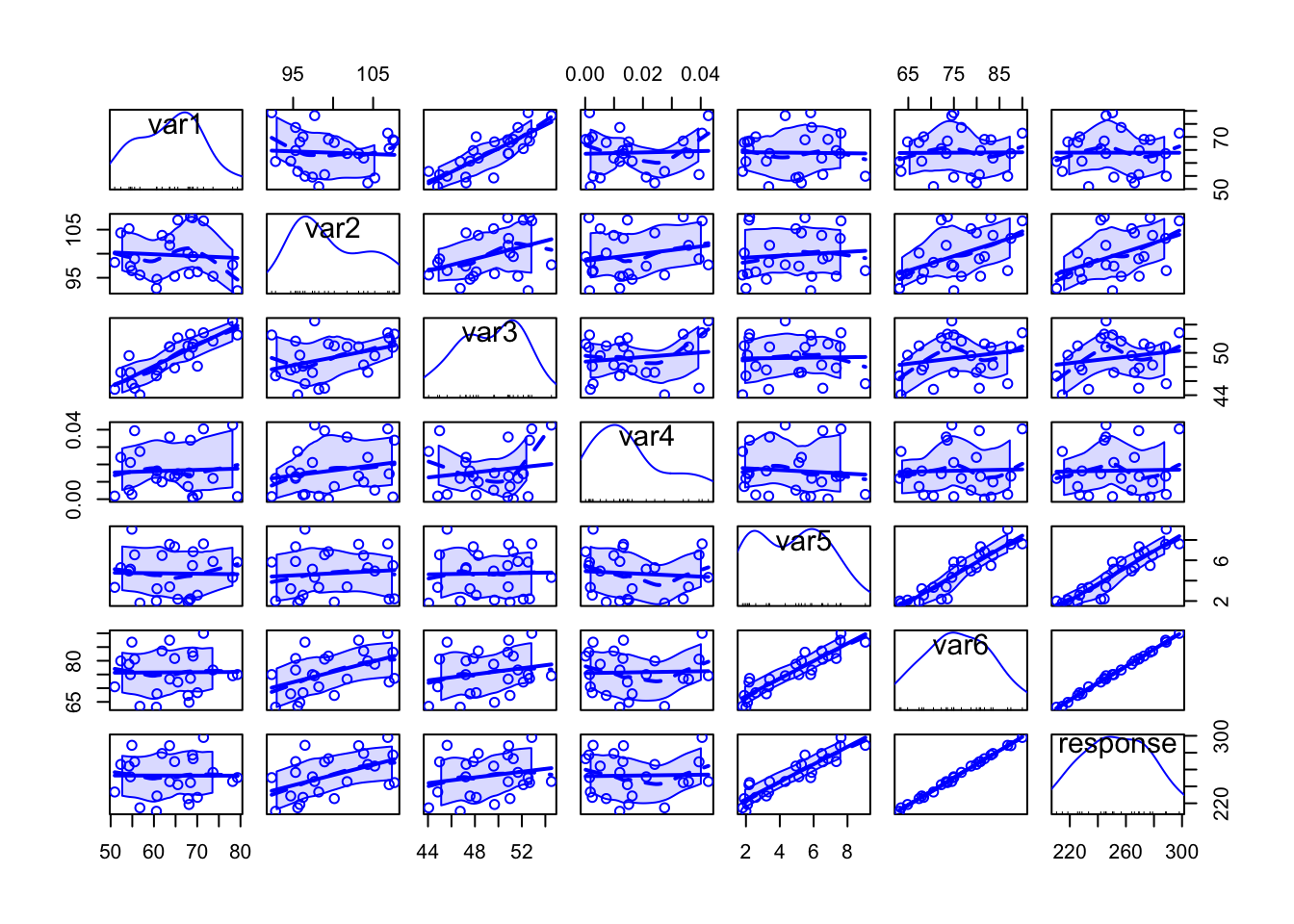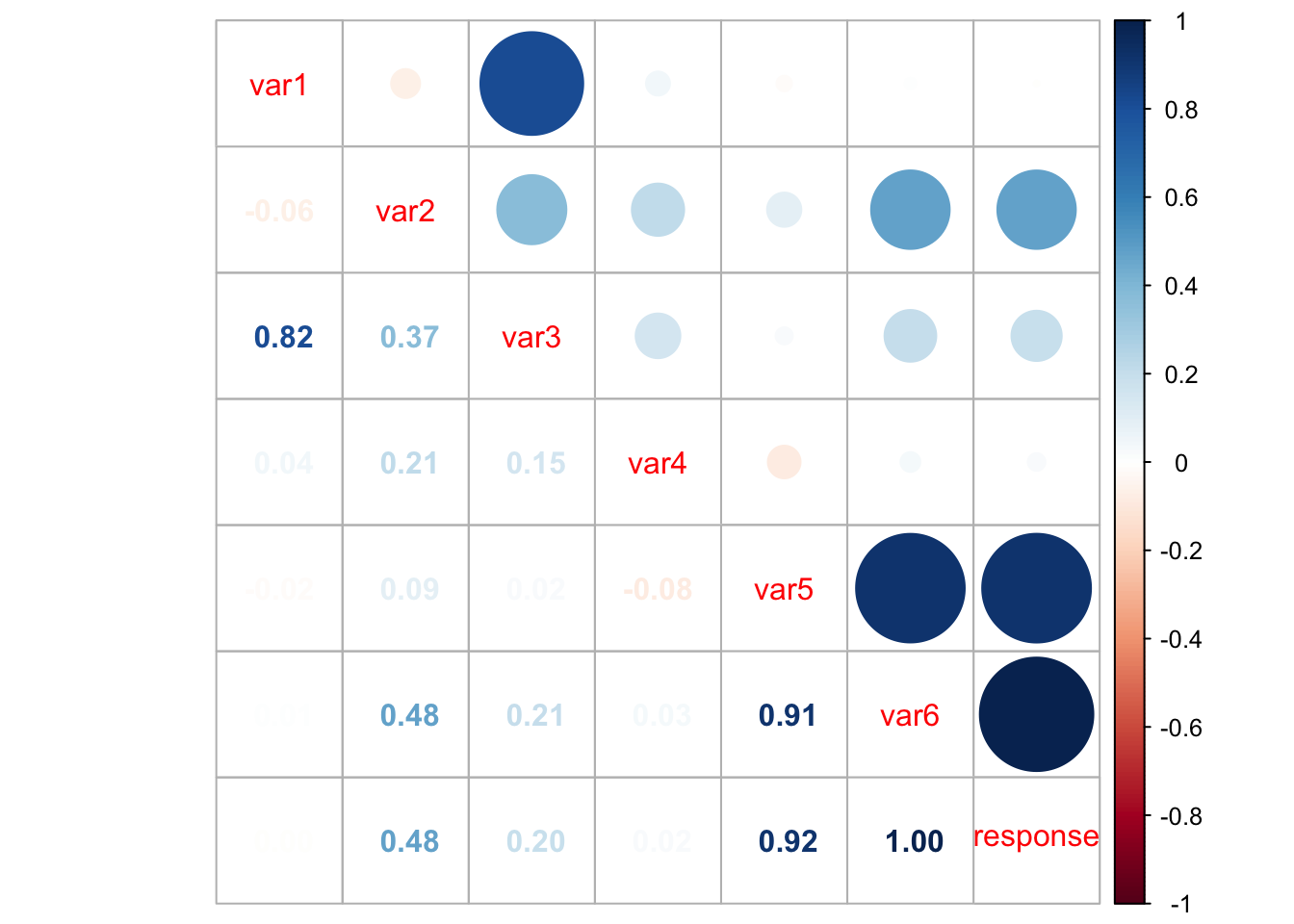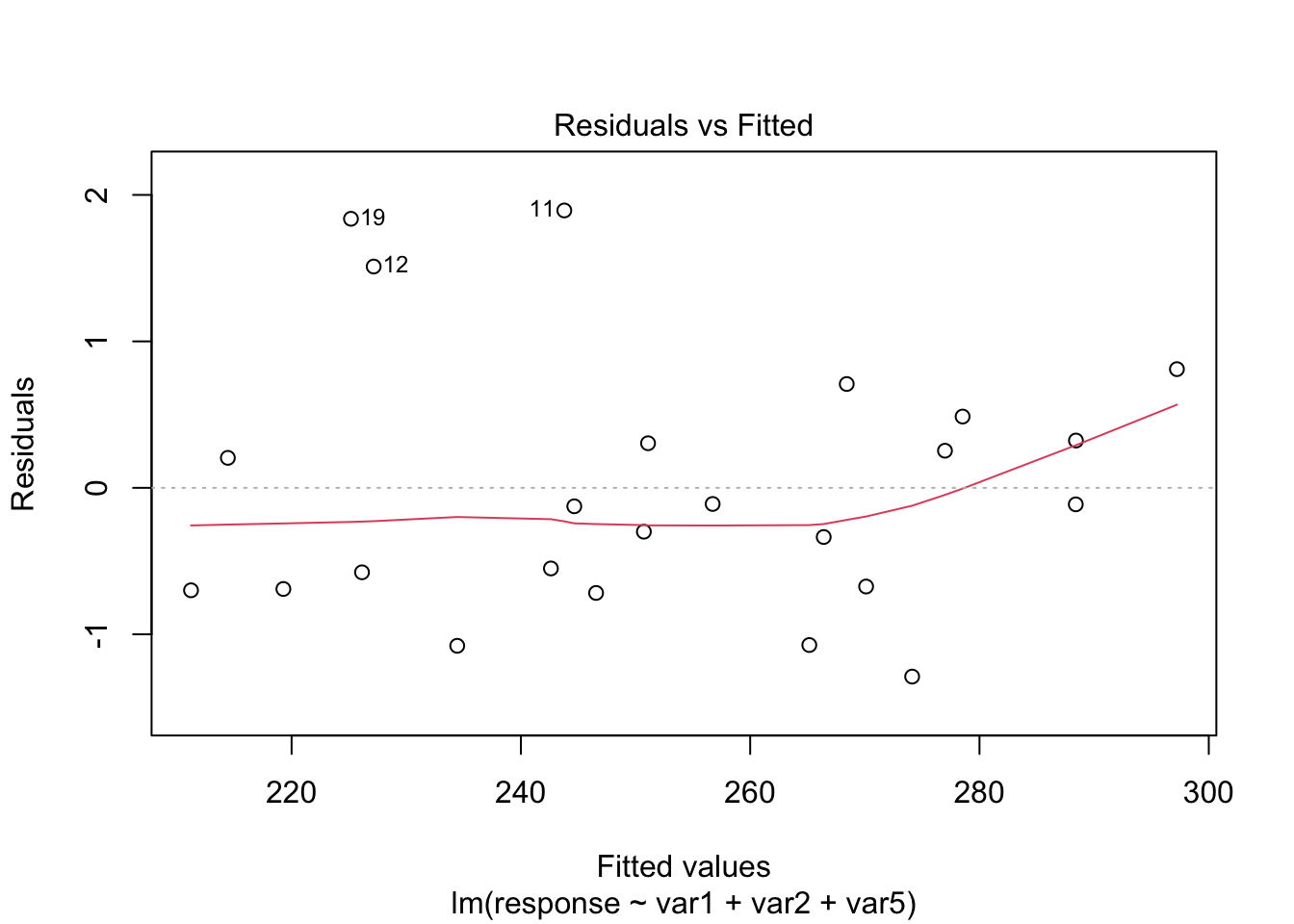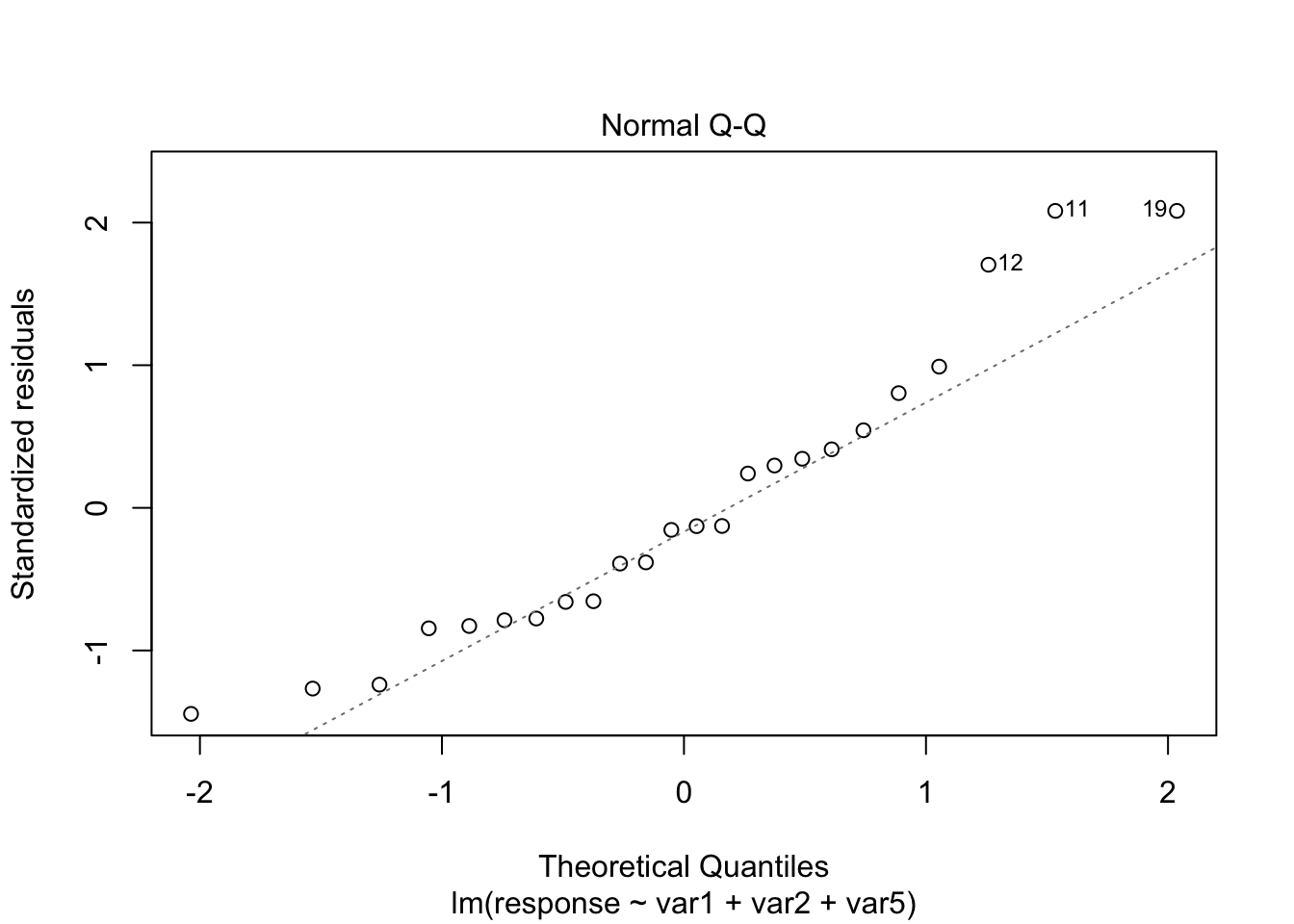# Objectives

In this case study, we will fit a multiple linear regression model. However, different than before, we will work with more variables. To do so, we will check for correlation among covariates, see how to perform variable selection, fit a model, check the assumptions, interpret it, and predict (and plot) new values based on this fitted model. When in higher dimension, visualization is difficult (and unnecessary for most cases).

# 1 Introduction

For this problem, we will analyse a data set with 6 variable (1 response variable + 6 covariates). Although their meaning may not be stated, we will see how important feature selection is when performing multiple regression analysis. You can download the .csv file here. The data set contains the following variables:

1. var1 (quantitative)
2. var2 (quantitative)
3. var3 (quantitative)
4. var4 (quantitative)
5. var5 (quantitative)
6. var6 (quantitative)
7. response (quantitative)

# 2 Exploring the data set

In order to explore the data set and perform initial analyses, we have to read it (with R) first. Provided that the .csv file is saved within the datasets/ folder, one can read the file in the following way.

col.names <- c('var1', 'var2', 'var3', 'var4', 'var5', 'var6', 'response')
data <- read.csv(file = 'datasets/data.csv', header = FALSE, sep = ',', col.names = col.names)
head(data, 5)
##       var1      var2     var3        var4     var5     var6 response
## 1 68.10730  95.83754 49.66851 0.015061421 2.090953 64.83720 218.5916
## 2 78.18420  97.69040 54.51643 0.042649961 4.320810 74.54103 245.8415
## 3 54.24527 105.20130 49.59829 0.005194938 4.948731 78.74680 264.0839
## 4 54.56271  97.41171 47.21550 0.021132252 5.127075 74.95861 251.3954
## 5 56.75478  95.57443 44.05604 0.027485738 1.801114 63.39468 214.6450

Now, let’s see a summary of our data.

summary(data)
##       var1            var2             var3            var4
##  Min.   :50.94   Min.   : 92.32   Min.   :44.06   Min.   :0.0001482
##  1st Qu.:56.45   1st Qu.: 95.77   1st Qu.:47.24   1st Qu.:0.0045800
##  Median :64.21   Median : 98.57   Median :49.63   Median :0.0131564
##  Mean   :63.96   Mean   : 99.73   Mean   :49.29   Mean   :0.0163943
##  3rd Qu.:68.94   3rd Qu.:103.94   3rd Qu.:51.37   3rd Qu.:0.0249283
##  Max.   :79.30   Max.   :107.66   Max.   :54.52   Max.   :0.0426500
##       var5            var6          response
##  Min.   :1.801   Min.   :63.08   Min.   :210.5
##  1st Qu.:2.486   1st Qu.:69.98   1st Qu.:232.2
##  Median :5.038   Median :74.97   Median :250.9
##  Mean   :4.709   Mean   :75.77   Mean   :252.8
##  3rd Qu.:6.493   3rd Qu.:81.05   3rd Qu.:270.3
##  Max.   :9.055   Max.   :90.00   Max.   :298.0

There are no missing values so that we can jump in into the exploratory analyses. However, since we want to use most information from this data set, it is not easy to visualize how strength can be written as a function of more than two variables at the same time. But it might be useful to see how variables are correlated. To do this, we can use the scatterplotMatrix() function from the car package, and the corrplot.mixed() function from the corrplot package.

library('car')

scatterplotMatrix(data)Specially when there are too many variables or too many data points per plot, it might be difficult to analyse all the details, but from the above plot we can have a rough idea on how each variable can be written as a function of others.

library('corrplot')

corrplot.mixed(cor(data))However, from the above plot we may have clearer information about the correlation between pair of variables. For instance, var1 and var3 are highly correlated, as well as var5 and var6, var5 and response, and var6 and response. This information can help us having an idea on which attributes better explain the dependent variable.

# 3 Fitting a model

Our very first task will be fitting a model with all variables so that we can try to explain how the response variable relates to the covariates. We can do this in the following way.

model <- lm(formula = response ~ ., data = data)
summary(model)
##
## Call:
## lm(formula = response ~ ., data = data)
##
## Residuals:
##      Min       1Q   Median       3Q      Max
## -1.29614 -0.41203 -0.05714  0.32644  1.68448
##
## Coefficients:
##             Estimate Std. Error t value Pr(>|t|)
## (Intercept) -0.01567    4.31348  -0.004   0.9971
## var1         0.16437    0.05915   2.779   0.0129 *
## var2         1.65363    0.25551   6.472 5.74e-06 ***
## var3        -0.24772    0.15677  -1.580   0.1325
## var4        15.25598   13.40879   1.138   0.2710
## var5         7.85702    1.19346   6.583 4.65e-06 ***
## var6         0.69112    0.38603   1.790   0.0912 .
## ---
## Signif. codes:  0 '***' 0.001 '**' 0.01 '*' 0.05 '.' 0.1 ' ' 1
##
## Residual standard error: 0.8177 on 17 degrees of freedom
## Multiple R-squared:  0.9992, Adjusted R-squared:  0.9989
## F-statistic:  3540 on 6 and 17 DF,  p-value: < 2.2e-16

From the above summary table, we may see two covariates that might not be significant, namely var3, var4, and var6. As we prefer simpler models over more complex models, provided they have the same performance, let’s remove the one with the highest p-value first (var4). We can do this using the update() function.

model2 <- update(model, ~. - var4)
summary(model2)
##
## Call:
## lm(formula = response ~ var1 + var2 + var3 + var5 + var6, data = data)
##
## Residuals:
##      Min       1Q   Median       3Q      Max
## -1.56817 -0.37178 -0.02967  0.30375  1.88355
##
## Coefficients:
##             Estimate Std. Error t value Pr(>|t|)
## (Intercept) -0.23242    4.34438  -0.053   0.9579
## var1         0.15298    0.05877   2.603   0.0180 *
## var2         1.57953    0.24908   6.341 5.64e-06 ***
## var3        -0.22602    0.15687  -1.441   0.1668
## var5         7.46733    1.15258   6.479 4.29e-06 ***
## var6         0.81454    0.37349   2.181   0.0427 *
## ---
## Signif. codes:  0 '***' 0.001 '**' 0.01 '*' 0.05 '.' 0.1 ' ' 1
##
## Residual standard error: 0.8244 on 18 degrees of freedom
## Multiple R-squared:  0.9991, Adjusted R-squared:  0.9989
## F-statistic:  4179 on 5 and 18 DF,  p-value: < 2.2e-16

Now, let’s remove var3.

model3 <- update(model2, ~. - var3)
summary(model3)
##
## Call:
## lm(formula = response ~ var1 + var2 + var5 + var6, data = data)
##
## Residuals:
##     Min      1Q  Median      3Q     Max
## -1.6041 -0.4214 -0.0859  0.4792  1.5974
##
## Coefficients:
##             Estimate Std. Error t value Pr(>|t|)
## (Intercept) -0.78392    4.44833  -0.176   0.8620
## var1         0.07899    0.02937   2.690   0.0145 *
## var2         1.46614    0.24293   6.035 8.33e-06 ***
## var5         7.19347    1.16854   6.156 6.46e-06 ***
## var6         0.90352    0.37864   2.386   0.0276 *
## ---
## Signif. codes:  0 '***' 0.001 '**' 0.01 '*' 0.05 '.' 0.1 ' ' 1
##
## Residual standard error: 0.8474 on 19 degrees of freedom
## Multiple R-squared:  0.999,  Adjusted R-squared:  0.9988
## F-statistic:  4944 on 4 and 19 DF,  p-value: < 2.2e-16

Although var6 has a p-value of 0.0276 and we already know that it is highly correlated with var5, let’s keep it for now. However, in order to have sufficiently simpler models, we can also compute and analyse the Variance Inflation Factor (VIF), which is a measure of the amount of multicollinearity in a set of multiple regression variables. According to this page the VIF for a regression model variable is equal to the ratio of the overall model variance to the variance of a model that includes only that single independent variable. This ratio is calculated for each independent variable. A high VIF indicates that the associated independent variable is highly collinear with the other variables in the model. Also, as a rule of thumb, we can exclude variables with VIF greater than 2, provided we do this for one variable at a time. To do this, we can use the vif() function from the car package.

vif(model3)
##       var1       var2       var5       var6
##   1.740913  44.823922 211.048276 271.824355

As we expected, var6 can be excluded from our model.

model4 <- update(model3, ~. - var6)
vif(model4)
##     var1     var2     var5
## 1.004377 1.012391 1.008489
summary(model4)
##
## Call:
## lm(formula = response ~ var1 + var2 + var5, data = data)
##
## Residuals:
##     Min      1Q  Median      3Q     Max
## -1.2887 -0.6780 -0.1195  0.3639  1.8939
##
## Coefficients:
##             Estimate Std. Error t value Pr(>|t|)
## (Intercept) -5.49457    4.42946  -1.240    0.229
## var1         0.12457    0.02479   5.026 6.48e-05 ***
## var2         2.03923    0.04057  50.268  < 2e-16 ***
## var5         9.97519    0.08976 111.135  < 2e-16 ***
## ---
## Signif. codes:  0 '***' 0.001 '**' 0.01 '*' 0.05 '.' 0.1 ' ' 1
##
## Residual standard error: 0.9416 on 20 degrees of freedom
## Multiple R-squared:  0.9988, Adjusted R-squared:  0.9986
## F-statistic:  5337 on 3 and 20 DF,  p-value: < 2.2e-16

Alternatively, we can do this model selection procedure according to another criteria, namely Akaike Information Criterion (AIC), which is used for evaluating how well a model fits the data it was generated from. AIC is used to compare different possible models and determine which one is the best fit for the data (reference). In R, we can use the stepAIC() function from the MASS package to automatize this procedure.

library('MASS')

(modelAIC <- stepAIC(model, direction = 'both'))
## Start:  AIC=-3.93
## response ~ var1 + var2 + var3 + var4 + var5 + var6
##
##        Df Sum of Sq    RSS     AIC
## - var4  1    0.8656 12.233 -4.1737
## <none>              11.368 -3.9349
## - var3  1    1.6697 13.037 -2.6458
## - var6  1    2.1433 13.511 -1.7894
## - var1  1    5.1645 16.532  3.0539
## - var2  1   28.0073 39.375 23.8818
## - var5  1   28.9812 40.349 24.4681
##
## Step:  AIC=-4.17
## response ~ var1 + var2 + var3 + var5 + var6
##
##        Df Sum of Sq    RSS     AIC
## <none>              12.233 -4.1737
## + var4  1    0.8656 11.368 -3.9349
## - var3  1    1.4109 13.644 -3.5540
## - var6  1    3.2324 15.466 -0.5465
## - var1  1    4.6053 16.839  1.4947
## - var2  1   27.3292 39.562 21.9958
## - var5  1   28.5272 40.760 22.7117
##
## Call:
## lm(formula = response ~ var1 + var2 + var3 + var5 + var6, data = data)
##
## Coefficients:
## (Intercept)         var1         var2         var3         var5         var6
##     -0.2324       0.1530       1.5795      -0.2260       7.4673       0.8145
summary(modelAIC)
##
## Call:
## lm(formula = response ~ var1 + var2 + var3 + var5 + var6, data = data)
##
## Residuals:
##      Min       1Q   Median       3Q      Max
## -1.56817 -0.37178 -0.02967  0.30375  1.88355
##
## Coefficients:
##             Estimate Std. Error t value Pr(>|t|)
## (Intercept) -0.23242    4.34438  -0.053   0.9579
## var1         0.15298    0.05877   2.603   0.0180 *
## var2         1.57953    0.24908   6.341 5.64e-06 ***
## var3        -0.22602    0.15687  -1.441   0.1668
## var5         7.46733    1.15258   6.479 4.29e-06 ***
## var6         0.81454    0.37349   2.181   0.0427 *
## ---
## Signif. codes:  0 '***' 0.001 '**' 0.01 '*' 0.05 '.' 0.1 ' ' 1
##
## Residual standard error: 0.8244 on 18 degrees of freedom
## Multiple R-squared:  0.9991, Adjusted R-squared:  0.9989
## F-statistic:  4179 on 5 and 18 DF,  p-value: < 2.2e-16
vif(modelAIC)
##       var1       var2       var3       var5       var6
##   7.366028  49.794159   6.922008 216.948426 279.464063

However, notice that stepAIC() only removed var4, and when we compute the VIF, we see that we can still have problems with multicollinearity (which might generate high variance of the estimated coefficients). Now, removing the variables with high VIF, we have

modelAIC2 <- update(modelAIC, ~. - var6)
vif(modelAIC2)
##     var1     var2     var3     var5
## 5.832034 2.231948 6.732781 1.008490
modelAIC3 <- update(modelAIC2, ~. - var3)
vif(modelAIC3)
##     var1     var2     var5
## 1.004377 1.012391 1.008489

Which is the same model as before. We will take this as our minimal model.

However, we still have to do a residual analysis. For doing this, we will do the “Residuals vs Fitted” and “Normal Q-Q” plots and run the appropriate tests, as before.

plot(model4, which = c(1, 2))From the plots, the assumptions of equal variance and normality for the residuals seem to hold. However, as fewer data points make the visual analysis difficult, it is also important to run the tests, namely, ncvTest() and shapiro.test() for the residuals (resid()).

ncvTest(model4)
## Non-constant Variance Score Test
## Variance formula: ~ fitted.values
## Chisquare = 1.481062, Df = 1, p = 0.22361
shapiro.test(resid(model4))
##
##  Shapiro-Wilk normality test
##
## data:  resid(model4)
## W = 0.93153, p-value = 0.1055

From the tests results, we fail to reject the null hypotheses—meaning that there is no evidence from the data that the assumptions of equal variance and normality for the residuals do not hold.

Our final model is

\begin{align*} \texttt{response}_i &= -5.495 + 0.125\texttt{var1}_i + 2.039\texttt{var2}_i + 9.975\texttt{var5}_i \end{align*}

# 4 Predicting unknown values

Now that we have a “good” fitted model, we can predict the value of response for new values of var1, var2, and var5. For instance, we can predict the value of response, such that var1, var2 and var5 are equal to 55, 100, and 70, respectively. We can also include a confidence and a prediction interval.

newdata <- data.frame(var1 = 55, var2 = 100, var5 = 70)

(pred1 <- predict(object = model4, newdata = newdata, interval = 'confidence'))
##        fit      lwr      upr
## 1 903.5433 891.3115 915.7751
(pred2 <- predict(object = model4, newdata = newdata, interval = 'prediction'))
##        fit      lwr      upr
## 1 903.5433 891.1548 915.9318